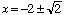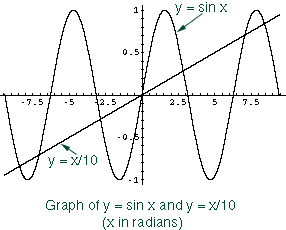Name: amandeep grover Who is asking: Teacher Level: Secondary Question: solve the equation sin x = x/10 Hi Amandeep, I like this question because it makes us think about what we mean by "solve". When we ask students to solve x2 + 4x + 2 = 0 we expect them to use the general quadratic and simplify to getFor sin x = x/10 I know one solution, x = 0 and from the diagram I know there are 6 more but I don't know a nice way to exprress them.I can approximate the solutions to as many decimal places as you might want but I can't express the solutions in a simple algebraic or trigonometric exprression. Cheers, Harley Go to Math Central